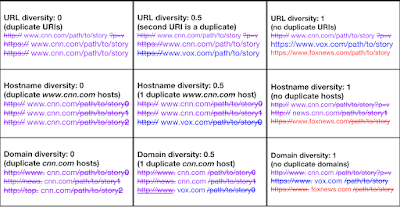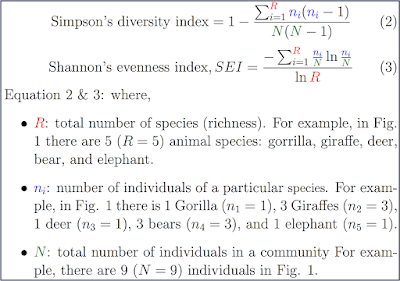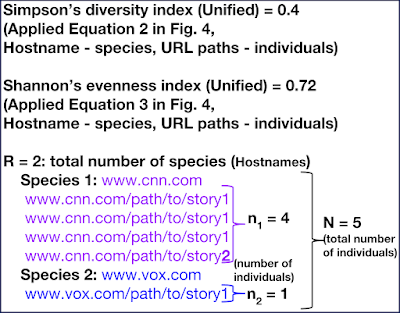### 2018-05-04: An exploration of URL diversity measures

Recently, as part of a research effort to describe a collections of URLs, I was faced with the problem of identifying a quantitative measure that indicates how many different kinds of URLs there are in a collection. In other words, what is the level of diversity in a collection of URLs? Ideally a diversity measure should produce a normalized value between 0 and 1. A 0 value means no diversity, for example, a collection of duplicate URLs (Fig. 2 first row, first column). In contrast, a diversity value of 1 indicates maximum diversity - all different URLs (Fig. 2, first row, last column):
1. http://www.cnn.com/path/to/story?p=v
2. https://www.vox.com/path/to/story
3. https://www.foxnews.com/path/to/story
Surprisingly, I did not find a standard URL diversity measure in the Web Science community, so I introduced the WSDL diversity index (described below). I acknowledge there may be other URL diversity measures in the Web Science community that exist under different names.
Not surprisingly, Biologist (especially Conservation Biologist) have multiple measures for quantifying biodiversity called diversity indices. In this blog post, I will briefly describe how some common biodiversity measures in addition to the WSDL diversity index can be used to quantify URL diversity. Additionally, I have provided recommendations for choosing a URL diversity measure depending on the problem domain. I have also provided a simple python script that reads a text file containing URLs and produces the URL diversity scores of the different measures introduced in this post.Fig. 2: WSDL URL diversity matrix of examples across multiple policies (URL, hostname, and domain). For all policies, the schemes, URL parameters, and fragments are stripped before calculation. For hostname diversity calculation, only the host is considered, and for domain diversity calculation, only the domain is considered.
I believe the problem of quantify how many different species there are in biological community is very similar to the problem of quantify how many different URLs there are in a collection of URLs. Biodiversity measures (or diversity indices) express the degree of variety in a community. Such measures answer questions such as: does a community of mushrooms only include one, two, or three species of mushrooms? Similarly, a URL diversity measure expresses the degree of variety in a collection of URLs and answers questions such as: does a collection of URLs only represent one (e.g cnn.com), two (cnn.com and foxnews.com), or three (cnn.com, foxnews.com, and nytimes.com) domains. Even though the biodiversity diversity indices and URL diversity measures are similar, it is important to note that since both domains are different their respective diversity measures reflect these differences. For example, the WSDL diversity index I introduce later does not reward duplicate URLs because duplicate URLs do not increase the informational value of a URL collection.

URL Diversity Measures (Source code)

Let us consider the WSDL diversity index for quantifying URL diversity, and apply popular biodiversity indices to quantify URL diversity.

URL preprocessing:
Since URLs have aliases, the following steps were taken before the URL diversity was calculated.

1. Scheme removal: This transforms
http://www.cnn.com/path/to/story?param1=value1&param2=value2#1
to
www.cnn.com/path/to/story?param1=value1&param2=value2#1

2. URL parameters and fragment removal: This transforms
www.cnn.com/path/to/story?param1=value1&param2=value2#1
to
www.cnn.com/path/to/story

3. Multi-policy and combined (or unified) policy URL diversity: For the WSDL diversity index (introduced below), the URL diversity can be calculated for multiple separate policies such as the URL (www.cnn.com/path/to/story), Domain (cnn.com), or Hostname (www.cnn.com). For the biodiversity measures introduced,  the URL diversity can also be calculated by combining policies. For example, URL diversity calculation done by combining Hostname (or domain) with URL paths. This involves considering the Hostnames (or domains) as the species and the URL paths as individuals. I call this combined policy approach of calculating URL diversity, unified diversity.

WSDL diversity index:

The WSDL diversity index (Fig. 3) rewards variety and not duplication. It is the ratio of unique items  (URIs or Domain names, or Hostnames) to the total number of items |C|. We subtract 1 from both numerator and denominator in order to normalize (0 - 1 range) the index. A value of 0 (e.g., Fig 2. first row, first column) is assigned by a list of duplicate URLs. A value of 1 is assigned by a list of distinct URLs (e.g., Fig. 2 first row, last column).Fig. 3: The WSDL diversity index (Equation 1) and the explanation of variables. U represents the count of unique URLs (or species - R).  |C| represents the number of URLs (or individuals N).
Unlike the other biodiversity indices introduced next, the WSDL diversity index can be calculated for separate policies: URL, Domain, and Hostname. This is because the numerator of the formula considers uniqueness not counts. In other words the numerator operates over sets of URLs (no duplicates allowed) unlike the biodiversity measures that operate over lists (duplicates allowed). Since the biodiversity measures introduced below take counts (count of species) into account, calculation of all the URL diversity across multiple policies results in the same diversity value except if the polices are combined (e.g., Hostname combined with URL paths).

The Simpson's diversity index (Fig. 4, equation 2) is a common diversity measure in Ecology that quantifies the degree of biodiversity (variety of species) in a community of organisms. It is also known as the Herfindahl–Hirschman index (HHI) in Economics, and Hunter-Gaston index in Microbiology. The index simultaneous quantifies two quantities - the richness (number of different kinds of organisms) and evenness (the proportion of each species present) in a bio-community. Additionally, the index produces diversity values ranging between 0 and 1. 0 means no diversity and 1 means maximum diversity.Fig. 4: Simpson's diversity index (Equation 2) and Shannon's evenness index (Equation 3) and the explanation of variables (R, n_i (n subscript i), and N) they share.
Applying the Simpson's diversity index to measure URL diversity:
There are multiple variants of the Simpson's diversity index, the variant showed in Fig. 4, equation. 2 is applicable to measuring URL diversity in two ways. First, we may consider URLs as the species of biological organisms (Method 1). Second, we may consider the Hostnames as the species (Method 2)  and the URL paths as the individuals. There are three parameters needed to use Simpson's diversity index (Fig. 4):
Method 1:
1. R - total number of species (or URLs)
2. n_i (n subscript i) - number of individuals for a given species, and
3. N - total number of individuals
Method 2 (Unified diversity):
1. R - total number of species where the Hostnames (or Domains) are the species
2. n_i (n subscript i) - number of individuals (URL paths) for a given species, and
3. N - total number of individuals
Fig. 5a applies Method 1 to calculate the URL diversity. In Fig. 5a, there are 3 different URLs interpreted as 3 species (R = 3) in the Simpson's diversity index formula (Fig. 4, equation. 2):
1. www.cnn.com/path/to/story1
2. www.cnn.com/path/to/story2
3. www.vox.com/path/to/story1Fig. 5a: Example showing how the Simpson's diversity index and Shannon's evenness index can be applied to calculate URL diversity by setting three variables: R represents the number of species (URLs). In the example, there are 3 different URLs. n_i (n subscript i) represents the count of the species (n_1 = 3, n_2 = 1, and n_3 = 1). N represents the total number of individuals (URLs). The Simpson's diversity index (Fig. 4, equation 2) is 0.7, Shannon's evenness index - 0.86
The first URL has 3 copies which can be interpreted as 3 individuals (for the first species - n_0) in the Simpson's diversity index formula. The second and third URLs have 1 copy each, similarly, this can be interpreted as 1 individual for the second (n_1) and third species (n_2). In total (including duplicates) we have 5 URL individuals (N = 5). With all the parameters of the Simpson's diversity index (Fig. 4, equation 2) set, the diversity index for the example in Fig. 5a is 0.7.Fig. 5b: Example showing how to the Simpson's diversity index and Shannon's diversity index can be applied to calculate unified URL diversity by interpreting Hostnames as the species (R) and the URLs paths as the individuals (n_i). This method combines the Hostname (or Domain) with URL paths for URL diversity calculation.
Fig. 5b applies Method 2 to calculate the Unified diversity. In the unified diversity calculation, the policies are combined (Hostname with URL paths). For example, in Fig. 5b the species represent the Hostnames and the URL paths are considered the individuals.

Shannon-Wiener diversity index:

The Shannon-Wiener diversity index or Shannon's diversity index comes from information theory where it is used to quantify the entropy in a string. However, in Ecology, similar to the Simpson's index, it is applied to quantify the biodiversity in a community. It simultaneously measures the richness (number of species) and the evenness (homogeneity of the species). The Shannon's Evenness Index  (SEI) is the Shannon's diversity index divided by the maximum diversity (ln(R)) which occurs when each species has the same frequency (maximum evenness).

Applying the SEI to measure URL diversity:Fig. 6: Example showing how the URL diversity indices differ. For example, the WSDL diversity index rewards URL uniqueness and penalizes URL duplication since the duplication of URLs does not increase informational value, but the Shannon's evenness index rewards balance in the proportion of URLs. It is also important to note that calculation of URL diversity across multiple separate policies (URL, domain, and hostname) is only possible with the WSDL diversity index.
The variables in the SEI are the same variables in the Simpson's diversity index. Fig 5a. evaluates the SEI (Equation 3) for a set of URLs, while Fig. 5b. calculates the unified URL diversity by interpreting the Hostnames as species.
I recommend using the WSDL diversity index for measuring URL diversity if the inclusion of a duplicate URL should not be rewarded and there is a need to calculate URL diversity across multiple separate policies (URL, domain, and hostname). Both Simpson's diversity index and Shannon evenness index strive to simultaneously capture richness and evenness. I believe Shannon's evenness index does a better job capturing evenness which happens when the proportion of species is distributed evenly (Fig. 6 first row, second column). I recommend using the Simpson's diversity and Shannon's evenness indices for URL diversity calculation when the definition of diversity is similar to the Ecological meaning of diversity and the presence of duplicate URLs need not penalize the overall diversity score. The source code that implements the URL diversity measures introduced here is publicly available.
-- Nwala (@acnwala)